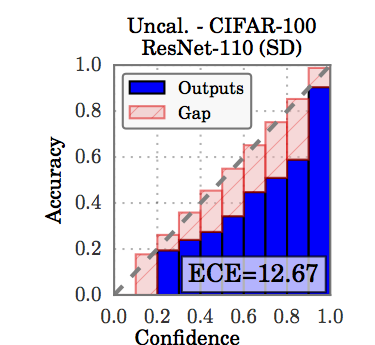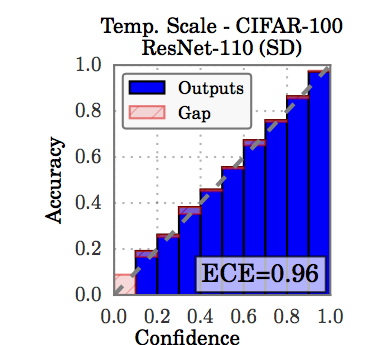# gpleiss / temperature_scaling

A simple way to calibrate your neural network.
Python

## Latest commit

Latest commit a474b23 Aug 31, 2018

## Files

Type Name Latest commit message Commit time
Failed to load latest commit information.models Aug 31, 2018.gitignore Aug 4, 2017LICENSE Aug 3, 2017README.md Aug 5, 2017demo.py Aug 31, 2018setup.cfg Aug 4, 2017temperature_scaling.py Aug 31, 2018train.py Aug 31, 2018

# Temperature Scaling

A simple way to calibrate your neural network. The `temperature_scaling.py` module can be easily used to calibrated any trained model.

Based on results from On Calibration of Modern Neural Networks.

## Motivation

TLDR: Neural networks tend to output overconfident probabilities. Temperature scaling is a post-processing method that fixes it.

Long:

Neural networks output "confidence" scores along with predictions in classification. Ideally, these confidence scores should match the true correctness likelihood. For example, if we assign 80% confidence to 100 predictions, then we'd expect that 80% of the predictions are actually correct. If this is the case, we say the network is calibrated.

A simple way to visualize calibration is plotting accuracy as a function of confidence. Since confidence should reflect accuracy, we'd like for the plot to be an identity function. If accuracy falls below the main diagonal, then our network is overconfident. This happens to be the case for most neural networks, such as this ResNet trained on CIFAR100.Temperature scaling is a post-processing technique to make neural networks calibrated. After temperature scaling, you can trust the probabilities output by a neural network:Temperature scaling divides the logits (inputs to the softmax function) by a learned scalar parameter. I.e.

``````softmax = e^(z/T) / sum_i e^(z_i/T)
``````

where `z` is the logit, and `T` is the learned parameter. We learn this parameter on a validation set, where T is chosen to minimize NLL.

## Demo

First train a DenseNet on CIFAR100, and save the validation indices:

`python train.py --data <path_to_data> --save <save_folder_dest>`

Then temperature scale it

`python demo.py --data <path_to_data> --save <save_folder_dest>`

## To use in a project

Copy the file `temperature_scaling.py` to your repo. Train a model, and save the validation set. (You must use the same validation set for training as for temperature scaling). You can do something like this:

```from temperature_scaling import ModelWithTemperature

orig_model = ... # create an uncalibrated model somehow
valid_loader = ... # Create a DataLoader from the SAME VALIDATION SET used to train orig_model

scaled_model = ModelWithTemperature(orig_model)